# Recurrent Neural Networks¶

Last time, we began tackling the problem of predicting the sentiment of tweets based on its text. We used GloVe embeddings, and summed up the embedding of each word in a tweet to obtain a representation of the tweet. We then built a model to predict the tweet's sentiment based on its representation.

One of the drawbacks of the previous approach is that the order of words is lost. The tweets "the cat likes the dog" and "the dog likes the cat" would have the exact same embedding, even though the sentences have different meanings.

Today, we wil use a recurrent neural network. We will treat each tweet as a sequence of words. Like before, we will use GloVe embeddings as inputs to the recurrent network. (As a sidenote, not all recurrent neural networks use word embeddings as input. If we had a small enough vocabulary, we could have used a one-hot embedding of the words.)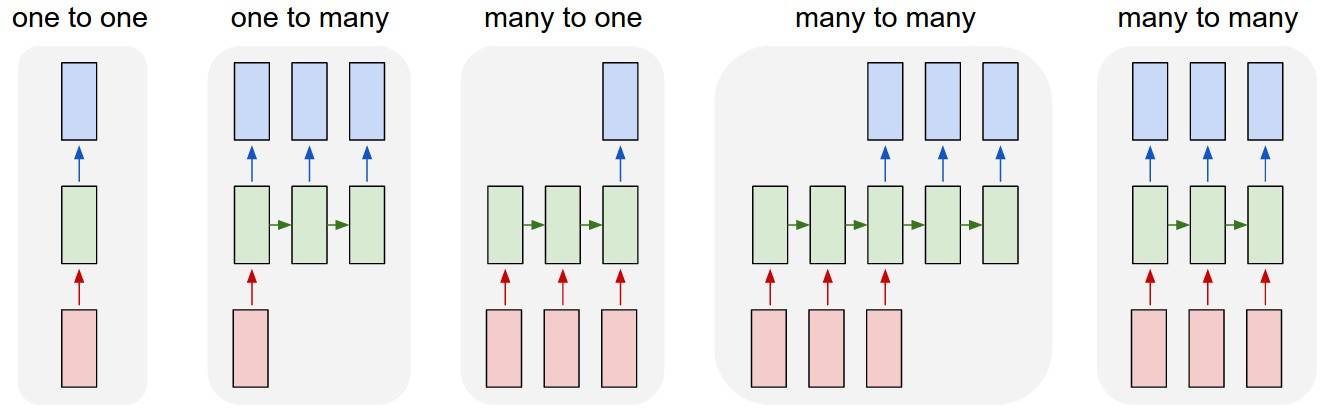In :
import csv
import torch
import torch.nn as nn
import torch.nn.functional as F
import torch.optim as optim
import torchtext
import numpy as np
import matplotlib.pyplot as plt

def get_data():
return csv.reader(open("training.1600000.processed.noemoticon.csv", "rt", encoding="latin-1"))

def split_tweet(tweet):
# separate punctuations
tweet = tweet.replace(".", " . ") \
.replace(",", " , ") \
.replace(";", " ; ") \
.replace("?", " ? ")
return tweet.lower().split()

glove = torchtext.vocab.GloVe(name="6B", dim=50, max_vectors=10000) # use 10k most common words


Since we are going to store the individual words in a tweet, we will defer looking up the word embeddings. Instead, we will store the index of each word in a PyTorch tensor. Our choice is the most memory-efficient, since it takes fewer bits to store an integer index than a 50-dimensional vector or a word.

In :
def get_tweet_words(glove_vector):
train, valid, test = [], [], []
for i, line in enumerate(get_data()):
if i % 29 == 0:
tweet = line[-1]
idxs = [glove_vector.stoi[w]        # lookup the index of word
for w in split_tweet(tweet)
if w in glove_vector.stoi] # keep words that has an embedding
if not idxs: # ignore tweets without any word with an embedding
continue
idxs = torch.tensor(idxs) # convert list to pytorch tensor
label = torch.tensor(int(line == "4")).long()
if i % 5 < 3:
train.append((idxs, label))
elif i % 5 == 4:
valid.append((idxs, label))
else:
test.append((idxs, label))
return train, valid, test

train, valid, test = get_tweet_words(glove)


Here's what an element of the training set looks like:

In :
tweet, label = train
print(tweet)
print(label)

tensor([   2,   11,    1,    7,    2,   81,  405,  684, 9912,    3,  245,  122,
4,   88,   20,    2,   89, 1968])
tensor(0)


Unlike in the past, each element of the training set will have a different shape. The difference will present some difficulties when we discuss batching later on.

In :
for i in range(10):
tweet, label = train[i]
print(tweet.shape)

torch.Size()
torch.Size()
torch.Size()
torch.Size()
torch.Size()
torch.Size()
torch.Size()
torch.Size()
torch.Size()
torch.Size()


## Embedding¶

We are also going to use an nn.Embedding layer, instead of using the variable glove directly. The reason is that the nn.Embedding layer lets us look up the embeddings of multiple words simultaneously.

In :
glove_emb = nn.Embedding.from_pretrained(glove.vectors)

# Example: we use the forward function of glove_emb to lookup the
# embedding of each word in tweet
tweet_emb = glove_emb(tweet)
tweet_emb.shape

Out:
torch.Size([31, 50])

## Recurrent Neural Network Module¶

PyTorch has variations of recurrent neural network modules. These modules computes the following:

$$hidden = updatefn(hidden, input)$$ $$output = outputfn(hidden)$$

These modules are more complex and less intuitive than the usual neural network layers, so let's take a look:

In :
rnn_layer = nn.RNN(input_size=50,    # dimension of the input repr
hidden_size=50,   # dimension of the hidden units
batch_first=True) # input format is [batch_size, seq_len, repr_dim]


Now, let's try and run this untrained rnn_layer on tweet_emb. We will need to add an extra dimension to tweet_emb to account for batching. We will also need to initialize a set of hidden units of size [batch_size, 1, repr_dim], to be used for the first set of computations.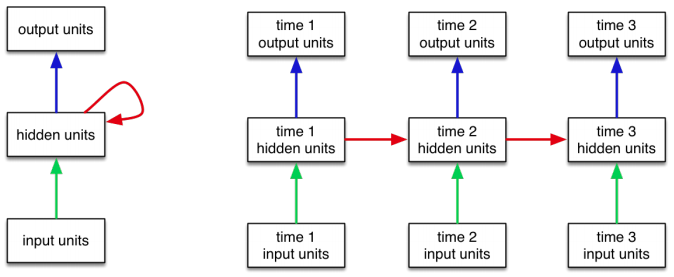In :
tweet_input = tweet_emb.unsqueeze(0) # add the batch_size dimension
h0 = torch.zeros(1, 1, 50)           # initial hidden state
out, last_hidden = rnn_layer(tweet_input, h0)


We don't technically have to explictly provide the initial hidden state, if we want to use an initial state of zeros. Just for today, we will be explicit about the hidden states that we provide.

In :
out2, last_hidden2 = rnn_layer(tweet_input)


Now, let's look at the output and hidden dimensions that we have:

In :
print(out.shape)
print(last_hidden.shape)

torch.Size([1, 31, 50])
torch.Size([1, 1, 50])


The shape of the hidden units is the same as our initial h0. The variable out, though, has the same shape as our input. The variable contains the concatenation of all of the output units for each word (i.e. at each time point).

Normally, we only care about the output at the final time point, which we can extract like this.

In :
out[:,-1,:]

Out:
tensor([[-0.4039, -0.4911,  0.6974,  0.5357,  0.2865,  0.5422,  0.1051, -0.3778,
0.1605,  0.3412, -0.2674,  0.0958, -0.3387,  0.1324, -0.2312,  0.3039,
-0.3610,  0.0963,  0.1394, -0.5885,  0.5903, -0.0425, -0.0825, -0.1130,
-0.2001,  0.2951, -0.4210, -0.3456,  0.1870, -0.1978, -0.2911, -0.7271,
-0.0278,  0.5637,  0.3253,  0.6454,  0.8467,  0.2044,  0.1874, -0.6023,
-0.4028,  0.3417,  0.0541, -0.2516,  0.0110, -0.4559,  0.4032,  0.1327,
-0.7200,  0.6882]], grad_fn=<SliceBackward>)

This tensor summarizes the entire tweet, and can be used as an input to a classifier.

## Building a Model¶

Let's put both the embedding layer, the RNN and the classifier into one model:

In :
class TweetRNN(nn.Module):
def __init__(self, input_size, hidden_size, num_classes):
super(TweetRNN, self).__init__()
self.emb = nn.Embedding.from_pretrained(glove.vectors)
self.hidden_size = hidden_size
self.rnn = nn.RNN(input_size, hidden_size, batch_first=True)
self.fc = nn.Linear(hidden_size, num_classes)

def forward(self, x):
# Look up the embedding
x = self.emb(x)
# Set an initial hidden state
h0 = torch.zeros(1, x.size(0), self.hidden_size)
# Forward propagate the RNN
out, _ = self.rnn(x, h0)
# Pass the output of the last time step to the classifier
out = self.fc(out[:, -1, :])
return out

model = TweetRNN(50, 50, 2)


Now, this model has a very similar API as the previous. We should be able to train this model similar to any other model that we have trained before. However, there is one caveat that we have been avoiding this entire time, batching.

## Batching¶

Unfortunately, we will not be able to use DataLoader with a batch_size of greater than one. This is because each tweet has a different shaped tensor.

In :
for i in range(10):
tweet, label = train[i]
print(tweet.shape)

torch.Size()
torch.Size()
torch.Size()
torch.Size()
torch.Size()
torch.Size()
torch.Size()
torch.Size()
torch.Size()
torch.Size()


PyTorch implementation of DataLoader class expects all data samples to have the same shape. So, if we create a DataLoader like below, it will throw an error when we try to iterate over its elements.

In :
#will_fail = torch.utils.data.DataLoader(train, batch_size=128)
#for elt in will_fail:
#    print("ok")


So, we will need a different way of batching.

One strategy is to pad shorter sequences with zero inputs, so that every sequence is the same length. The following PyTorch utilities are helpful.

• torch.nn.utils.rnn.pad_sequence
• torch.nn.utils.rnn.pad_packed_sequence
• torch.nn.utils.rnn.pack_sequence
• torch.nn.utils.rnn.pack_padded_sequence

(Actually, there are more powerful helpers in the torchtext module that we will use in Lab 5. We'll stick to these in this demo, so that you can see what's actually going on under the hood.)

In :
from torch.nn.utils.rnn import pad_sequence

tweet_padded = pad_sequence([tweet for tweet, label in train[:10]],
batch_first=True)

Out:
torch.Size([10, 31])

Now, we can pass multiple tweets in a batch through the RNN at once!

In :
out = model(tweet_padded)
out.shape

Out:
torch.Size([10, 2])

One issue we overlooked was that in our TweetRNN model, we always take the last output unit as input to the final classifier. Now that we are padding the input sequences, we should really be using the output at a previous time step. Recurrent neural networks therefore require much more record keeping than MLPs or even CNNs.

There is yet another problem: the longest tweet has many, many more words than the shortest. Padding tweets so that every tweet has the same length as the longest tweet is impractical. Padding tweets in a mini-batch, however, is much more reasonable.

In practice, practitioners will batch together tweets with the same length. For simplicity, we will do the same. We will implement a (more or less) straightforward way to batch tweets. Our implementation will be flawed, and we will discuss these flaws.

In :
import random

class TweetBatcher:
def __init__(self, tweets, batch_size=32, drop_last=False):
# store tweets by length
self.tweets_by_length = {}
for words, label in tweets:
# compute the length of the tweet
wlen = words.shape
# put the tweet in the correct key inside self.tweet_by_length
if wlen not in self.tweets_by_length:
self.tweets_by_length[wlen] = []
self.tweets_by_length[wlen].append((words, label),)

#  create a DataLoader for each set of tweets of the same length
tweets,
batch_size=batch_size,
shuffle=True,
drop_last=drop_last) # omit last batch if smaller than batch_size
for wlen, tweets in self.tweets_by_length.items()}

def __iter__(self): # called by Python to create an iterator
# make an iterator for every tweet length
while iters:
# pick an iterator (a length)
im = random.choice(iters)
try:
yield next(im)
except StopIteration:
# no more elements in the iterator, remove it
iters.remove(im)


Let's take a look at our batcher in action. We will set drop_last to be true for training, so that all of our batches have exactly the same size.

In :
for i, (tweets, labels) in enumerate(TweetBatcher(train, drop_last=True)):
if i > 5: break
print(tweets.shape, labels.shape)

torch.Size([32, 32]) torch.Size()
torch.Size([32, 11]) torch.Size()
torch.Size([32, 7]) torch.Size()
torch.Size([32, 11]) torch.Size()
torch.Size([32, 23]) torch.Size()
torch.Size([32, 4]) torch.Size()


Just to verify that our batching is reasonable, here is a modification of the get_accuracy function we wrote last time.

In :
def get_accuracy(model, data_loader):
correct, total = 0, 0
for tweets, labels in data_loader:
output = model(tweets)
pred = output.max(1, keepdim=True)
correct += pred.eq(labels.view_as(pred)).sum().item()
total += labels.shape
return correct / total

test_loader = TweetBatcher(test, batch_size=64, drop_last=False)

Out:
0.5002292526364053

Our training code will also be very similar to the code we wrote last time:

In :
def train_rnn_network(model, train, valid, num_epochs=5, learning_rate=1e-5):
criterion = nn.CrossEntropyLoss()
optimizer = torch.optim.Adam(model.parameters(), lr=learning_rate)
losses, train_acc, valid_acc = [], [], []
epochs = []
for epoch in range(num_epochs):
for tweets, labels in train:
pred = model(tweets)
loss = criterion(pred, labels)
loss.backward()
optimizer.step()
losses.append(float(loss))

epochs.append(epoch)
print("Epoch %d; Loss %f; Train Acc %f; Val Acc %f" % (
epoch+1, loss, train_acc[-1], valid_acc[-1]))
# plotting
plt.title("Training Curve")
plt.plot(losses, label="Train")
plt.xlabel("Epoch")
plt.ylabel("Loss")
plt.show()

plt.title("Training Curve")
plt.plot(epochs, train_acc, label="Train")
plt.plot(epochs, valid_acc, label="Validation")
plt.xlabel("Epoch")
plt.ylabel("Accuracy")
plt.legend(loc='best')
plt.show()


Let's train our model. Note that there will be some inaccuracies in computing the training loss. We are dropping some data from the training set by setting drop_last=True. Again, the choice is not ideal, but simplifies our code.

In :
model = TweetRNN(50, 50, 2)
train_loader = TweetBatcher(train, batch_size=64, drop_last=True)
valid_loader = TweetBatcher(valid, batch_size=64, drop_last=False)

Epoch 1; Loss 0.558688; Train Acc 0.648549; Val Acc 0.646164
Epoch 2; Loss 0.618095; Train Acc 0.663442; Val Acc 0.661564
Epoch 3; Loss 0.526807; Train Acc 0.666115; Val Acc 0.658905
Epoch 4; Loss 0.649005; Train Acc 0.665415; Val Acc 0.657530
Epoch 5; Loss 0.531141; Train Acc 0.663410; Val Acc 0.661931
Epoch 6; Loss 0.633337; Train Acc 0.670666; Val Acc 0.665139
Epoch 7; Loss 0.565262; Train Acc 0.671111; Val Acc 0.666147
Epoch 8; Loss 0.607091; Train Acc 0.676744; Val Acc 0.670272
Epoch 9; Loss 0.600520; Train Acc 0.668279; Val Acc 0.662297
Epoch 10; Loss 0.578448; Train Acc 0.680340; Val Acc 0.670364
Epoch 11; Loss 0.654185; Train Acc 0.674325; Val Acc 0.670547
Epoch 12; Loss 0.606876; Train Acc 0.681963; Val Acc 0.674214
Epoch 13; Loss 0.560580; Train Acc 0.680563; Val Acc 0.676139
Epoch 14; Loss 0.576739; Train Acc 0.686004; Val Acc 0.676872
Epoch 15; Loss 0.615441; Train Acc 0.686418; Val Acc 0.679989
Epoch 16; Loss 0.566258; Train Acc 0.687150; Val Acc 0.679989
Epoch 17; Loss 0.537208; Train Acc 0.692082; Val Acc 0.681089
Epoch 18; Loss 0.638282; Train Acc 0.689887; Val Acc 0.683656
Epoch 19; Loss 0.607846; Train Acc 0.691446; Val Acc 0.681364
Epoch 20; Loss 0.546912; Train Acc 0.692337; Val Acc 0.677239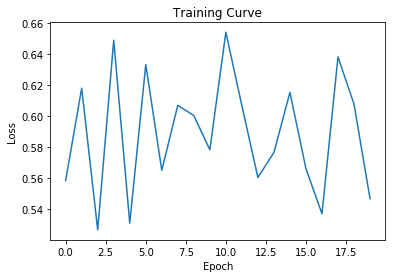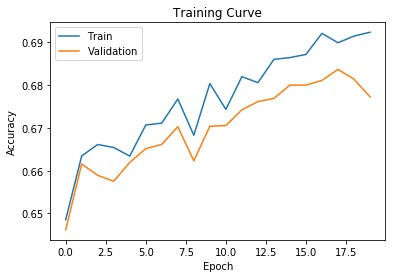Out:
0.6806969280146722

The hidden size and the input embedding size don't have to be the same.

In :
#model = TweetRNN(50, 100, 2)


## LSTM for Long-Term Dependencies¶

There are variations of recurrent neural networks that are more powerful. One such variation is the Long Short-Term Memory (LSTM) module. An LSTM is like a more powerful version of an RNN that is better at perpetuating long-term dependencies. Instead of having only one hidden state, an LSTM keeps track of both a hidden state and a cell state.

In :
lstm_layer = nn.LSTM(input_size=50,   # dimension of the input repr
hidden_size=50,   # dimension of the hidden units
batch_first=True) # input format is [batch_size, seq_len, repr_dim]


Remember the single tweet that we worked with earlier?

In :
tweet_emb.shape

Out:
torch.Size([31, 50])

This is how we can feed this tweet into the LSTM, similar to what we tried with the RNN earlier.

In :
tweet_input = tweet_emb.unsqueeze(0) # add the batch_size dimension
h0 = torch.zeros(1, 1, 50)     # initial hidden layer
c0 = torch.zeros(1, 1, 50)     # initial cell state
out, last_hidden = lstm_layer(tweet_input, (h0, c0))
out.shape

Out:
torch.Size([1, 31, 50])

So an LSTM version of our model would look like this:

In :
class TweetLSTM(nn.Module):
def __init__(self, input_size, hidden_size, num_classes):
super(TweetLSTM, self).__init__()
self.emb = nn.Embedding.from_pretrained(glove.vectors)
self.hidden_size = hidden_size
self.rnn = nn.LSTM(input_size, hidden_size, batch_first=True)
self.fc = nn.Linear(hidden_size, num_classes)

def forward(self, x):
# Look up the embedding
x = self.emb(x)
# Set an initial hidden state and cell state
h0 = torch.zeros(1, x.size(0), self.hidden_size)
c0 = torch.zeros(1, x.size(0), self.hidden_size)
# Forward propagate the LSTM
out, _ = self.rnn(x, (h0, c0))
# Pass the output of the last time step to the classifier
out = self.fc(out[:, -1, :])
return out

model_lstm = TweetLSTM(50, 50, 2)

Epoch 1; Loss 0.616468; Train Acc 0.696188; Val Acc 0.685306
Epoch 2; Loss 0.538515; Train Acc 0.695806; Val Acc 0.685947
Epoch 3; Loss 0.566302; Train Acc 0.696570; Val Acc 0.685397
Epoch 4; Loss 0.650394; Train Acc 0.696856; Val Acc 0.689889
Epoch 5; Loss 0.515213; Train Acc 0.697683; Val Acc 0.685947
Epoch 6; Loss 0.551632; Train Acc 0.697397; Val Acc 0.683839
Epoch 7; Loss 0.538383; Train Acc 0.697047; Val Acc 0.689156
Epoch 8; Loss 0.591978; Train Acc 0.696888; Val Acc 0.689981
Epoch 9; Loss 0.589179; Train Acc 0.698511; Val Acc 0.684022
Epoch 10; Loss 0.594392; Train Acc 0.698224; Val Acc 0.689156
Epoch 11; Loss 0.448012; Train Acc 0.698829; Val Acc 0.687597
Epoch 12; Loss 0.614235; Train Acc 0.699529; Val Acc 0.684664
Epoch 13; Loss 0.510262; Train Acc 0.700197; Val Acc 0.689889
Epoch 14; Loss 0.517869; Train Acc 0.699306; Val Acc 0.685397
Epoch 15; Loss 0.512688; Train Acc 0.697301; Val Acc 0.689797
Epoch 16; Loss 0.569831; Train Acc 0.699911; Val Acc 0.686131
Epoch 17; Loss 0.547411; Train Acc 0.700516; Val Acc 0.688606
Epoch 18; Loss 0.561993; Train Acc 0.699529; Val Acc 0.685214
Epoch 19; Loss 0.535264; Train Acc 0.699434; Val Acc 0.690347
Epoch 20; Loss 0.538262; Train Acc 0.700165; Val Acc 0.689522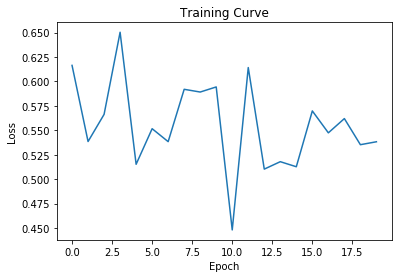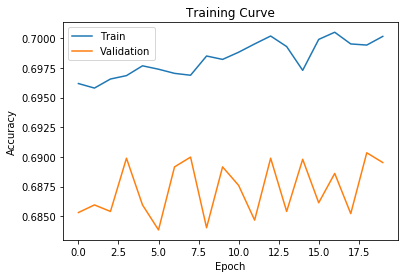Out:
0.6902338376891334

## GRU for Long-Term Dependencies¶

Another variation of an RNN is the Gated-Recurrent Unit (GRU). The GRU is invented after LSTM, and is intended to be a simplification of the LSTM that is still just as powerful. The nice thing about GRU units is that they have only one hidden state.

In :
gru_layer = nn.GRU(input_size=50,   # dimension of the input repr
hidden_size=50,   # dimension of the hidden units
batch_first=True) # input format is [batch_size, seq_len, repr_dim]


The GRU API is virtually identical to that of the vanilla RNN:

In :
tweet_input = tweet_emb.unsqueeze(0) # add the batch_size dimension
h0 = torch.zeros(1, 1, 50)     # initial hidden layer
out, last_hidden = gru_layer(tweet_input, h0)
out.shape

Out:
torch.Size([1, 31, 50])

So a GRU version of our model would look similar to before:

In :
class TweetGRU(nn.Module):
def __init__(self, input_size, hidden_size, num_classes):
super(TweetGRU, self).__init__()
self.emb = nn.Embedding.from_pretrained(glove.vectors)
self.hidden_size = hidden_size
self.rnn = nn.GRU(input_size, hidden_size, batch_first=True)
self.fc = nn.Linear(hidden_size, num_classes)

def forward(self, x):
# Look up the embedding
x = self.emb(x)
# Set an initial hidden state
h0 = torch.zeros(1, x.size(0), self.hidden_size)
# Forward propagate the GRU
out, _ = self.rnn(x, h0)
# Pass the output of the last time step to the classifier
out = self.fc(out[:, -1, :])
return out

model_lstm = TweetGRU(50, 50, 2)

Epoch 1; Loss 0.644203; Train Acc 0.701661; Val Acc 0.691172
Epoch 2; Loss 0.611624; Train Acc 0.700261; Val Acc 0.691081
Epoch 3; Loss 0.511547; Train Acc 0.701534; Val Acc 0.686131
Epoch 4; Loss 0.526848; Train Acc 0.701152; Val Acc 0.689981
Epoch 5; Loss 0.583295; Train Acc 0.702489; Val Acc 0.688056
Epoch 6; Loss 0.551774; Train Acc 0.701407; Val Acc 0.687139
Epoch 7; Loss 0.518153; Train Acc 0.700866; Val Acc 0.690531
Epoch 8; Loss 0.629933; Train Acc 0.702202; Val Acc 0.690531
Epoch 9; Loss 0.569499; Train Acc 0.702107; Val Acc 0.688514
Epoch 10; Loss 0.605321; Train Acc 0.701884; Val Acc 0.689797
Epoch 11; Loss 0.559082; Train Acc 0.701598; Val Acc 0.690256
Epoch 12; Loss 0.683046; Train Acc 0.702870; Val Acc 0.690531
Epoch 13; Loss 0.634333; Train Acc 0.703507; Val Acc 0.690072
Epoch 14; Loss 0.545534; Train Acc 0.703793; Val Acc 0.689431
Epoch 15; Loss 0.471686; Train Acc 0.703125; Val Acc 0.690897
Epoch 16; Loss 0.500653; Train Acc 0.704112; Val Acc 0.691356
Epoch 17; Loss 0.625901; Train Acc 0.704271; Val Acc 0.689431
Epoch 18; Loss 0.606249; Train Acc 0.704493; Val Acc 0.689889
Epoch 19; Loss 0.551744; Train Acc 0.705130; Val Acc 0.689889
Epoch 20; Loss 0.620697; Train Acc 0.704939; Val Acc 0.692364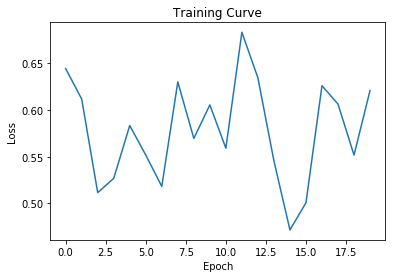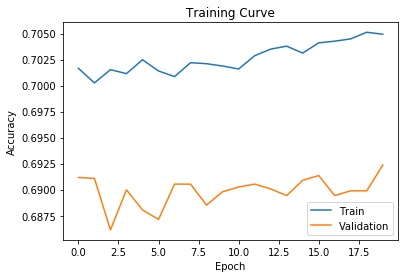Out:
0.6939935809261807